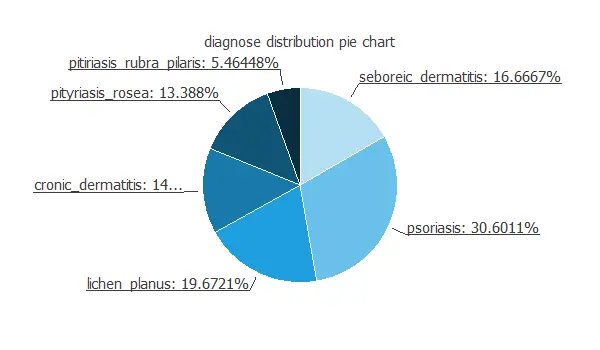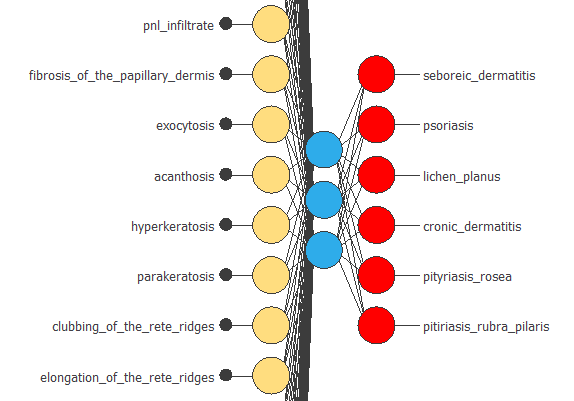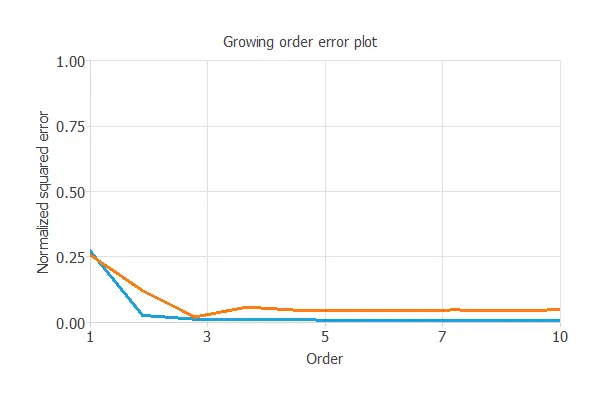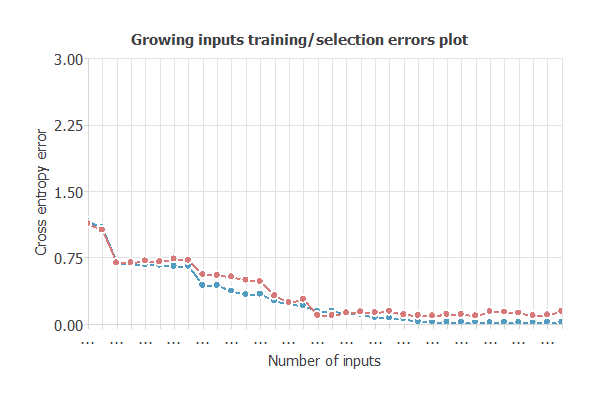In this example, we intend to identify the dermatologic disease that the patient is suffering. We do so based on clinical and histopathologic data.

The diagnosis of erythemato-squamous diseases (ESDs) is important in dermatology because they are pretty common and share clinical features with very few differences. This characteristic makes an accurate diagnosis a challenging problem as they have overlapping signs and symptoms. The diseases in this group include psoriasis, seborrheic dermatitis, lichen planus, and pityriasis rosea, among others. The development of a predictive tool can assist the physician in diagnosing the patient more effectively and quickly.

### Contents

This example is solved using Neural Designer. You can follow this example along using the free trial.

## 1. Application type

The variable we will predict is categorical, the type of ESDs (psoriasis, seborrheic dermatitis, etc.). Thus, this is a classification project.

We aim to model class membership probabilities conditioned on the input variables using artificial intelligence and machine learning.

## 2. Data set

The file dermatology.csv contains the data for this example. The number of rows (instances) in the data set is 366, and the number of columns (variables) is 35.

The number of input variables, or attributes for each sample, is 34. All input variables are numeric except for the target variable, diagnose, that corresponds to the type of ESDs (psoriasis, seborrheic dermatitis, lichen planus, pityriasis rosea, chronic dermatitis, or pityriasis rubra pilaris).

In the data set, the family history characteristic is 1 if there is a family history for any of these diseases; otherwise, it is 0. The age feature represents the age of the patient. Every other variable (clinical and histopathological) has a value in the range of 0 to 3. Here, 0 indicates that the feature was absent, 3 shows the most significant amount possible, and 1 or 2 means the relative intermediate values.

The following list summarizes the variables information:

• erythema: skin redness.
• scaling: scaly skin.
• definite_borders: clear, sharp border separating it from its surrounds.
• itching: unpleasant sensation on the skin that provokes the desire to rub or scratch the area.
• koebner_phenomenon: refers to when people with a specific dermatological disease manifest disease lesions in other skin lesions.
• polygonal_papules: presence of shiny, flat-topped, and firm on palpation circumscribed elevations.
• follicular_papules: presence of skin lesion, less than one centimeter in diameter, circumscribed, elevated, with well-defined borders and solid content
• oral_mucosal_involvement: presence of skin lesions inside the mouth.
• knee_and_elbow_involvement: skin lesions in the knee and/or the elbow.
• scalp_involvement: skin lesions in the scalp.
• family_history: (0 or 1).
• age: age of the patient in years.
• melanin_incontinence: spillage of melanin from the basal keratinocytes into the underlying connective tissue.
• eosinophils in the infiltrate: bone marrow-derived cells that infiltrate skin and mucous membranes.
• pnl_infiltrate: pure neuritic leprosy, no skin lesions but larger nerve trunks or their branches are enlarged, accompanied with a sensory loss in the areas.
• fibrosis_of_the_papillary_dermis: excess development of fibrous connective tissue in the papillary dermis.
• exocytosis: passage to the epidermis of cells foreign to it.
• acanthosis: Presence of dark, velvety skin areas in body creases.
• hyperkeratosis: thickening of the outer layer of the skin.
• parakeratosis: a mode of keratinization characterized by the retention of nuclei in the stratum corneum.
• clubbing_of_the_rete_ridges: the epithelial extensions that project into the underlying connective tissue in both skin and mucous membranes.
• elongation_of_the_rete_ridges: hyperpigmentation of the basal layer in the papillary dermis.
• thinning_of_the_suprapapillary_epidermis: a thinning of the granular layer at the tips of the papillae.
• spongiform_pustule: an epidermal pustule formed by infiltration of neutrophils into necrotic epidermis in pustular psoriasis.
• munro_microabcess: is an abscess in the stratum corneum of the epidermis due to the infiltration of neutrophils from papillary dermis into the epidermal stratum corneum.
• focal_hypergranulosis: is an increased thickness of the stratum granulosum.
• disappearance_of_the_granular layer: disappearance of the skin granular layer.
• vacuolisation_and_damage_of_basal_layer: presence of vacuolisation and damage of skin basal layer.
• spongiosis: presence of intercellular edema.
• saw_tooth_appearance_of_retes: appearance of saw tooth patterns under the skin tissue.
• follicular_horn_plug: presence of follicular horn plugs
• perifollicular_parakeratosis: keratinization characterized by the retention of nuclei in tissues surrounding skin follicles.
• inflammatory_mononuclear_inflitrate: increase in the number of infiltrating mononuclear cells in the skin.
• band_like_infiltrate: basal epidermis in a banded pattern.

The instances are split at random by Neural Designer into training (60%), selection (20%), and testing (20%) subsets. The user can modify these values to another ones if desired.

We can perform a few related analytics and check that the data is good.

We can calculate the data statistics and draw a table with descriptive statistics (minimums, maximums, means, and standard deviations) of all the variables in the data set.

We can also calculate the data distribution to see the number of instances belonging to each class in the data set.The number of instances belonging to psoriasis is 30.6%, most of the samples. On the other hand, pityriasis rubra pilaris represent approximately 5.5%, with the smallest number of instances.

Finally, we can calculate the inputs-targets correlations, that might indicate to us which factors most influence the diagnosis for each type of skin lesion.Here, the most correlated variables with the diagnose are follicular horn plug, follicular papules and elongation of the rete ridges.

## 3. Neural network

The second step is to set a neural network to represent the classification function, that for this class of applications is composed of:

The scaling layer contains the statistics on the inputs calculated from the data file and the method for scaling the input variables. Here the minimum-maximum method has been set. The scaling layer has 34 inputs since there are 34 input variables.

A perceptron layer with a hidden hyperbolic tangent layer. As a starting point, we use three neurons in the hidden layer.

The probabilistic layer contains the method to interpret the outputs as probabilities. The activation function used is the Softmax, as we have a categorical variable with more than two possibilities. The probabilistic layer has three inputs. It also has one output per category, representing the probability of a sample being in that category.

The following figure is a representation of this neural network:Since we have a vast range of inputs, please note that the previous figure is cropped for better visualization, as the original image has a considerable height.

## 4. Training strategy

The procedure used in the learning process is called a training strategy. The training strategy is applied to the neural network to obtain the best possible performance. The type of training is determined by how the adjustment of the parameters in the neural network takes place. This strategy is composed of two terms:

• A loss index.
• An optimization algorithm.

The loss index is the normalized squared error with L2 regularization.

Finding a neural network that minimizes the loss index is the machine learning problem. That is, a neural network that fits the data set (error term) and does not oscillate (regularization term).

The optimization algorithm that we use is the quasi-Newton method. This is also the standard optimization algorithm for multiple classification problems.

The following chart shows how the error decreases with the iterations during the training process:Both curves’ behavior is similar along with the iterations, which means that no over-fitting has appeared. The final training and selection errors are training error = 0.01 WSE and selection error = 0.09 WSE, respectively. This indicates that the neural network has good generalization capabilities.

## 5. Model selection

The objective of model selection is to find the network architecture with the best generalization properties (that minimizes the error on the selected instances of the data set).

We want to find a neural network with a selection error of less than 0.09 WSE, which is the value we have achieved so far.

Model selection algorithms train several network architectures with a different number of neurons and select that with the smallest selection error.

The neural selection method starts with a small number of neurons and increases the complexity at each iteration. The following chart shows the training error (blue) and the selection error (orange) as a function of the number of neurons.It shows that neurons selection does not decrease the training error a lot. But still, we obtain a minor error rate with four neurons.

We also can perform input selection. Input selection algorithms automatically extract those features in the data set that provide the best generalization capabilities. That is, they search for the subset of inputs that minimizes the selection error.After executing this algorithm, we get the optimal inputs number of 30. We have 4 unused input variables. With this number of inputs we further reduce the selection error = 0.03 WSE. We obtain a significantly better model and less complex. The number of hidden neurons represents the complexity, which is 30: 3: 6.

## 6. Testing analysis

Once we have trained the model, we perform a testing analysis to validate its prediction capacity. We use a subset of data that has not been used before, the testing instances.

The next table shows the confusion matrix for our problem. The rows represent the real classes in the confusion matrix, and the columns are the predicted classes for the testing data.

Predicted seboreic_dermatitis Predicted psoriasis Predicted lichen_planus Predicted cronic_dermatitis Predicted pityriasis_rosea Predicted pitiriasis_rubra_pilaris
Real seboreic_dermatitis 10 (13.7%) 0 0 0 1 (1.4%) 0
Real psoriasis 0 24 (32.9%) 0 0 0 0
Real lichen_planus 0 0 15 (20.5%) 0 0 0
Real cronic_dermatitis 0 0 0 11 (15.1%) 0 0
Real pityriasis_rosea 0 0 0 0 9 (12.3%) 0
Real pityriasis_rubra_pilaris 0 0 0 0 0 3 (4.1%)

The number of instances that the model can correctly predict is 72 (98.6%), while it misclassifies only 1 (1.4%).
This shows that our predictive model has an excellent classification accuracy.

## 7. Model deployment

The neural network is now able to predict the activity of new people in the so-called model deployment phase.

The file dermatology.py implements the mathematical expression of the neural network in Python. This script can be embedded in any tool to make predictions on new data.

Besides, we can use the mathematical expression of the neural network, which is listed next.

scaled_erythema = (erythema-2.068310022)/0.6638460159;
scaled_ scaling = ( scaling-1.795079947)/0.7005680203;
scaled_ definite_borders = ( definite_borders-1.549180031)/0.9062849879;
scaled_itching = (itching-1.366119981)/1.136739969;
scaled_koebner_phenomenon = (koebner_phenomenon-0.6338800192)/0.9067749977;
scaled_ polygonal_papules = ( polygonal_papules-0.4480870068)/0.9560170174;
scaled_ follicular_papules = ( follicular_papules-0.1666669995)/0.5698090196;
scaled_ oral_mucosal_involvement = ( oral_mucosal_involvement-0.3770489991)/0.8330060244;
scaled_knee_and_elbow_involvement = (knee_and_elbow_involvement-0.6147540212)/0.9816359878;
scaled_scalp_involvement = (scalp_involvement-0.519125998)/0.9044010043;
scaled_ family_history =  family_history*(1+1)/(1-(0))-0*(1+1)/(1-0)-1;
scaled_ melanin_incontinence = ( melanin_incontinence-0.4043720067)/0.8686280251;
scaled_ pnl_infiltrate = ( pnl_infiltrate-0.5464479923)/0.8143339753;
scaled_ fibrosis_of_the_papillary_dermis = ( fibrosis_of_the_papillary_dermis-0.3360660076)/0.8519740105;
scaled_ exocytosis = ( exocytosis-1.368849993)/1.102910042;
scaled_ parakeratosis = ( parakeratosis-1.289620042)/0.9163079858;
scaled_ clubbing_of_the_rete_ridges = ( clubbing_of_the_rete_ridges-0.6639339924)/1.055379987;
scaled_ elongation_of_the_rete_ridges = ( elongation_of_the_rete_ridges-0.9918029904)/1.160570025;
scaled_ thinning_of_the_suprapapillary_epidermis = ( thinning_of_the_suprapapillary_epidermis-0.6338800192)/1.03350997;
scaled_ spongiform_pustule = ( spongiform_pustule-0.2950820029)/0.6696590185;
scaled_ munro_microabcess = ( munro_microabcess-0.3633880019)/0.7586820126;
scaled_ focal_hypergranulosis = ( focal_hypergranulosis-0.3934429884)/0.8482459784;
scaled_ disappearance_of_the_granular_layer = ( disappearance_of_the_granular_layer-0.4644809961)/0.8637170196;
scaled_ vacuolisation_and_damage_of_basal_layer = ( vacuolisation_and_damage_of_basal_layer-0.4562839866)/0.9535660148;
scaled_ spongiosis = ( spongiosis-0.9535520077)/1.128630042;
scaled_ saw_tooth_appearance_of_retes = ( saw_tooth_appearance_of_retes-0.4535520077)/0.953437984;
scaled_ follicular_horn_plug = ( follicular_horn_plug-0.1038250029)/0.4498170018;
scaled_ perifollicular_parakeratosis = ( perifollicular_parakeratosis-0.1147539988)/0.4880549908;
scaled_band_like_infiltrate = (band_like_infiltrate-0.5546450019)/1.104390025;
scaled_ age = ( age-35.50270081)/nan;

perceptron_layer_1_output_0 = tanh( -0.997498 + (scaled_erythema*0.617432) + (scaled_ scaling*0.169983) + (scaled_ definite_borders*-0.0402832) + (scaled_itching*-0.299438) + (scaled_koebner_phenomenon*0.79187) + (scaled_ polygonal_papules*0.64563) + (scaled_ follicular_papules*0.493164) + (scaled_ oral_mucosal_involvement*-0.651794) + (scaled_knee_and_elbow_involvement*0.717834) + (scaled_scalp_involvement*0.420959) + (scaled_ family_history*0.0270386) + (scaled_ melanin_incontinence*-0.392029) + (scaled_ pnl_infiltrate*-0.970032) + (scaled_ fibrosis_of_the_papillary_dermis*-0.8172) + (scaled_ exocytosis*-0.271118) + (scaled_ parakeratosis*-0.705383) + (scaled_ clubbing_of_the_rete_ridges*-0.668213) + (scaled_ elongation_of_the_rete_ridges*0.97699) + (scaled_ thinning_of_the_suprapapillary_epidermis*-0.108643) + (scaled_ spongiform_pustule*-0.761841) + (scaled_ munro_microabcess*-0.990662) + (scaled_ focal_hypergranulosis*-0.982178) + (scaled_ disappearance_of_the_granular_layer*-0.244263) + (scaled_ vacuolisation_and_damage_of_basal_layer*0.0632935) + (scaled_ spongiosis*0.142334) + (scaled_ saw_tooth_appearance_of_retes*0.203491) + (scaled_ follicular_horn_plug*0.214294) + (scaled_ perifollicular_parakeratosis*-0.667542) + (scaled_band_like_infiltrate*0.32605) + (scaled_ age*-0.0984497) );
perceptron_layer_1_output_1 = tanh( 0.127136 + (scaled_erythema*-0.295776) + (scaled_ scaling*-0.885925) + (scaled_ definite_borders*0.215332) + (scaled_itching*0.566589) + (scaled_koebner_phenomenon*0.605164) + (scaled_ polygonal_papules*0.0397339) + (scaled_ follicular_papules*-0.396118) + (scaled_ oral_mucosal_involvement*0.751892) + (scaled_knee_and_elbow_involvement*0.453308) + (scaled_scalp_involvement*0.911743) + (scaled_ family_history*0.851379) + (scaled_ melanin_incontinence*0.0786743) + (scaled_ pnl_infiltrate*-0.715332) + (scaled_ fibrosis_of_the_papillary_dermis*-0.0758667) + (scaled_ exocytosis*-0.529358) + (scaled_ parakeratosis*0.724426) + (scaled_ clubbing_of_the_rete_ridges*-0.580811) + (scaled_ elongation_of_the_rete_ridges*0.559265) + (scaled_ thinning_of_the_suprapapillary_epidermis*0.687256) + (scaled_ spongiform_pustule*0.99353) + (scaled_ munro_microabcess*0.999329) + (scaled_ focal_hypergranulosis*0.222961) + (scaled_ disappearance_of_the_granular_layer*-0.215149) + (scaled_ vacuolisation_and_damage_of_basal_layer*-0.46759) + (scaled_ spongiosis*-0.405457) + (scaled_ saw_tooth_appearance_of_retes*0.680237) + (scaled_ follicular_horn_plug*-0.952515) + (scaled_ perifollicular_parakeratosis*-0.248291) + (scaled_band_like_infiltrate*-0.814758) + (scaled_ age*0.35437) );
perceptron_layer_1_output_2 = tanh( -0.613403 + (scaled_erythema*-0.887573) + (scaled_ scaling*-0.982422) + (scaled_ definite_borders*0.837524) + (scaled_itching*-0.448242) + (scaled_koebner_phenomenon*-0.454224) + (scaled_ polygonal_papules*0.175781) + (scaled_ follicular_papules*0.382324) + (scaled_ oral_mucosal_involvement*0.675171) + (scaled_knee_and_elbow_involvement*0.452942) + (scaled_scalp_involvement*-0.0301514) + (scaled_ family_history*-0.589294) + (scaled_ melanin_incontinence*0.487427) + (scaled_ pnl_infiltrate*-0.0631104) + (scaled_ fibrosis_of_the_papillary_dermis*-0.0841064) + (scaled_ exocytosis*0.898254) + (scaled_ parakeratosis*0.488831) + (scaled_ clubbing_of_the_rete_ridges*-0.783447) + (scaled_ elongation_of_the_rete_ridges*0.198059) + (scaled_ thinning_of_the_suprapapillary_epidermis*-0.229553) + (scaled_ spongiform_pustule*0.469971) + (scaled_ munro_microabcess*0.217896) + (scaled_ focal_hypergranulosis*0.144775) + (scaled_ disappearance_of_the_granular_layer*-0.277344) + (scaled_ vacuolisation_and_damage_of_basal_layer*-0.696899) + (scaled_ spongiosis*-0.549805) + (scaled_ saw_tooth_appearance_of_retes*-0.149719) + (scaled_ follicular_horn_plug*0.605713) + (scaled_ perifollicular_parakeratosis*0.0341797) + (scaled_band_like_infiltrate*0.979919) + (scaled_ age*0.503052) );

probabilistic_layer_combinations_0 = -0.308899 +0.00958252*perceptron_layer_1_output_0 -0.705017*perceptron_layer_1_output_1 +0.899109*perceptron_layer_1_output_2
probabilistic_layer_combinations_1 = -0.662048 -0.716858*perceptron_layer_1_output_0 +0.810181*perceptron_layer_1_output_1 +0.385742*perceptron_layer_1_output_2
probabilistic_layer_combinations_2 = 0.314575 -0.393921*perceptron_layer_1_output_0 -0.146912*perceptron_layer_1_output_1 -0.859253*perceptron_layer_1_output_2
probabilistic_layer_combinations_3 = -0.0162354 +0.933167*perceptron_layer_1_output_0 +0.366333*perceptron_layer_1_output_1 -0.693542*perceptron_layer_1_output_2
probabilistic_layer_combinations_4 = -0.872925 +0.754456*perceptron_layer_1_output_0 +0.643311*perceptron_layer_1_output_1 +0.164063*perceptron_layer_1_output_2
probabilistic_layer_combinations_5 = 0.399475 -0.61731*perceptron_layer_1_output_0 -0.644226*perceptron_layer_1_output_1 +0.634338*perceptron_layer_1_output_2

sum = exp(probabilistic_layer_combinations_0) + exp(probabilistic_layer_combinations_1) + exp(probabilistic_layer_combinations_2) + exp(probabilistic_layer_combinations_3) + exp(probabilistic_layer_combinations_4) + exp(probabilistic_layer_combinations_5);

seboreic_dermatitis = exp(probabilistic_layer_combinations_0)/sum;
psoriasis = exp(probabilistic_layer_combinations_1)/sum;
lichen_planus = exp(probabilistic_layer_combinations_2)/sum;
cronic_dermatitis = exp(probabilistic_layer_combinations_3)/sum;
pityriasis_rosea = exp(probabilistic_layer_combinations_4)/sum;
pitiriasis_rubra_pilaris = exp(probabilistic_layer_combinations_5)/sum;


## References

• The data for this problem has been taken from the UCI Machine Learning Repository.
• Nilsel Ilter, M.D., Ph.D.,Gazi University,School of Medicine.
• H. Altay Guvenir, PhD.,Bilkent University,Department of Computer Engineering and Information Science.